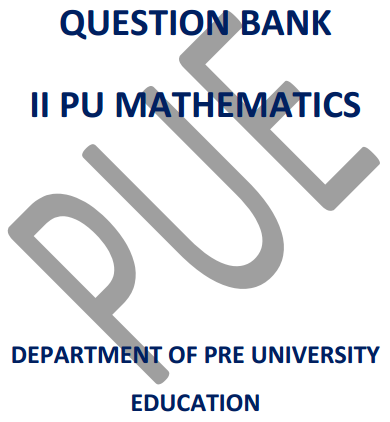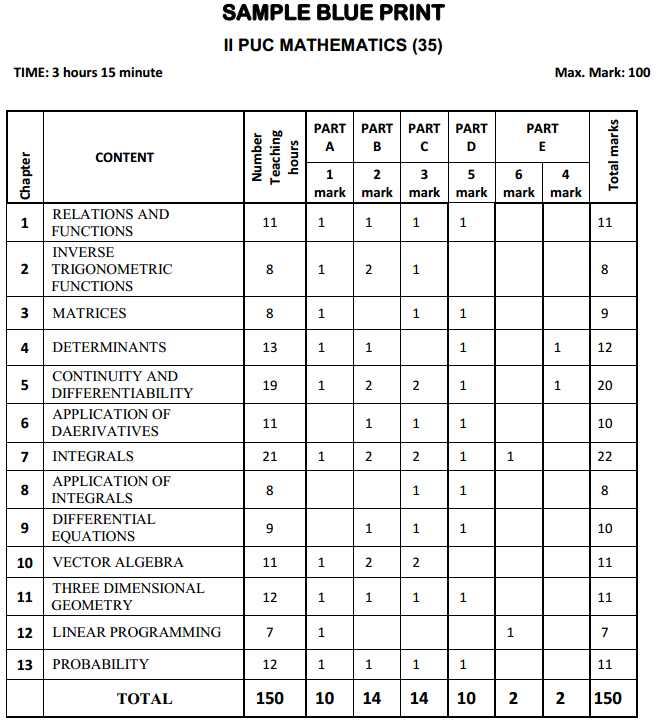# 2nd PUC Maths Question Bank with Answers Karnataka

Karnataka State Board Solutions for 2nd PUC Maths Question Banks with Answers, Solutions, Notes, Guide Pdf are prevailing here in direct pdf links for effective exam preparation. Students who are studying for 2nd PUC Maths textbook solutions can refer to this page for grabbing KSEEB 2nd PUC Maths Solutions pdf chapter wise for free. By reading the textbook solutions. You Can Download 2nd PUC Question Bank with Answers from Karnataka State Board solutions, you will be able to understand all the exercises and score good marks.

Also, there are so many advantages that you can avail from the Karnataka State Board Solutions for 2nd PUC Maths Question Bank with Answers, Solutions pdf. So download 2nd PUC Maths Notes from KTBS Solutions Pdf via quick links & access them easily while preparation. Students can also read 2nd PUC Maths Model Question Papers with Answers hope will definitely help for your board exams.## Karnataka 2nd PUC Maths Question Bank with Answers

Provided Karnataka State Board solutions for 2nd PUC Maths textbook solutions are prepared by subject experts after ample research. So students are suggested to download the 2nd PUC Maths Question Bank with Answers and Textbook Solutions from the available PDF links and kickstart your preparations for final exams. You can download them freely & practice regularly to score high marks in the examinations. Access the below Chapter-wise links and learn complete by using 2nd PUC Maths Question Bank with Answers from Karnataka State Board solutions pdf.

2nd PUC Maths Question Bank Chapter 1 Relations and Functions

2nd PUC Maths Question Bank Chapter 2 Inverse Trigonometric Functions

2nd PUC Maths Question Bank Chapter 3 Matrices

2nd PUC Maths Question Bank Chapter 4 Determinants

2nd PUC Maths Question Bank Chapter 5 Continuity and Differentiability

2nd PUC Maths Question Bank Chapter 6 Application of Derivatives

2nd PUC Maths Question Bank Chapter 7 Integrals

2nd PUC Maths Question Bank Chapter 8 Application of Integrals

2nd PUC Maths Question Bank Chapter 9 Differential Equations

2nd PUC Maths Question Bank Chapter 10 Vector Algebra

2nd PUC Maths Question Bank Chapter 11 Three Dimensional Geometry

2nd PUC Maths Question Bank Chapter 12 Linear Programming

2nd PUC Maths Question Bank Chapter 13 Probability

Karnataka 2nd PUC Maths Blue Print of Model Question Paper### 2nd PUC Maths Design of the Question Paper

MATHEMATICS (35)
CLASS : II PUC

Time: 3 hour 15 minute ; Max. Marks: 100
(of which 15 minute for reading the question paper).
The weightage of the distribution of marks over different dimensions of the question paper shall be as follows:

2nd PUC Maths Weightage to Objectives:

 Objective Weightage Marks Knowledge 40% 60/150 Understanding 30% 45/150 Application 20% 30/150 Skill 10% 15/150

2nd PUC Maths Weightage to level of difficulty:

 Level Weightage Marks Easy 35% 53/150 Average 55% 82/150 Difficult 10% 15/150

2nd PUC Maths Weightage to content/subject units:

 Chapter No. Chapter No. of teaching Hours Marks 1 RELATIONS AND FUNCTIONS 11 11 2 INVERSE TRIGONOMETRIC FUNCTIONS 8 8 3 MATRICES 8 9 4 DETERMINANTS 13 12 5 CONTINUITY AND DIFFERENTIABILITY 19 20 6 APPLICATION OF DERIVATIVES 11 10 7 INTEGRALS 21 22 8 APPLICATION OF INTEGRALS 8 8 9 DIFFERENTIAL EQUATIONS 9 10 10 VECTORS 11 11 11 THREE DIMENSIONAL GEOMETRY 12 11 12 LINEAR PROGRAMMING 7 7 13 PROBABILITY 12 11 TOTAL 150 150

2nd PUC Maths Pattern of the question paper:

 Part Type of questions Number of questions to be set Number of questions to be answered Remarks A 1 mark questions 10 10 Compulsory part B 2 mark questions 14 10 —– C 3 mark questions 14 10 —– D 5 mark questions 10 6 Questions must be asked from specific set of topics as mentioned below. E 10 mark questions (Each question with two sub divisions namely a) 6 mark and b) 4 mark ) 2 1

Instructions:
Content areas to select questions for PART – D and PART – E
a) In part D

1. Relations and functions: Problems on verification of invertibility of a function and writing its inverse.
2. Matrices: Problems on algebra of matrices .
3. Determinants: Problems on finding solution to simultaneous linear equations involving three unknown quantities by matrix method.
4. Continuity and differentiability: Problems on second derivatives only.
5. Application of derivatives: Problems on derivative as a rate measurer.
6. Integrals: Derivations on indefinite integrals and evaluation of an indefinite integral by using the derived formula.
7. Application of integrals: Problems on finding the area of the bounded region by the method of integration.
8. Differential equations: Problems on solving linear differential equations only.
9. Three dimensional geometry: Derivations on three dimensional geometry (both vector and Cartesian form)
10. Probability: Problems on Bernoulli Trials and Binomial distribution.

b) In PART E:
(i) 6 mark questions must be taken from the following content areas
Integrals: Derivations on definite integrals and evaluation of a definite integral using the derived formula.
Linear programming: Problems on linear programming.
(ii) 4 mark question must be taken from the following content areas.
Continuity and differentiability: Problems on continuous functions.
Determinants: Problems on evaluation of determinants by using properties.

We wish the data shed above will benefit you at the time of your preparation & gain good scores. So, download Karnataka State Board 2nd PUC Maths Question Bank with Answers from here and become a topper in the final examinations. Moreover, keep in touch with our site & get all updated chapter wise KSEEB State Board Solutions for all subjects.